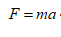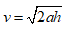# In an experiment, an object is released from rest near and above Earth’s surface. A student must determine the relationship between the direction of the gravitational force exerted on the object and the change in momentum caused by that force. What data could the student collect to determine the magnitude and direction of the gravitational force and the change in momentum of the object? Select two answers. *  (A) The mass of the object, because it is required to determine the force due to gravity exerted on the object, and the velocity of the object the instant before it reaches Earth’s surface, because it is required to determine the change in velocity of the object. (B) The mass of the object, because it is required to determine the force due to gravity exerted on the object, and the distance fallen by the object, because the force is exerted during the entire falling distance. (C) The velocity of the object the instant before it reaches Earth’s surface, because it is required to determine the change in velocity of the object, and the acceleration of the object, because it is required to determine the force due to gravity exerted on the object. (D) The distance fallen by the object, because the force is exerted during the entire falling distance, and the acceleration of the object, because it is required to determine the force due to gravity exerted on the object.

Question
50 views
In an experiment, an object is released from rest near and above Earth’s surface. A student must determine the relationship between the direction of the gravitational force exerted on the object and the change in momentum caused by that force. What data could the student collect to determine the magnitude and direction of the gravitational force and the change in momentum of the object? Select two answers. *

(A) The mass of the object, because it is required to determine the force due to gravity exerted on the object, and the velocity of the object the instant before it reaches Earth’s surface, because it is required to determine the change in velocity of the object.

(B) The mass of the object, because it is required to determine the force due to gravity exerted on the object, and the distance fallen by the object, because the force is exerted during the entire falling distance.

(C) The velocity of the object the instant before it reaches Earth’s surface, because it is required to determine the change in velocity of the object, and the acceleration of the object, because it is required to determine the force due to gravity exerted on the object.

(D) The distance fallen by the object, because the force is exerted during the entire falling distance, and the acceleration of the object, because it is required to determine the force due to gravity exerted on the object.
check_circle

Step 1

if the student assumes the gravitational acceleration is a constant value, then it is required only the mass of the object and the distance fallen by the object to determine the entire calculation.

thus, option (B) is only required.

the force is given byHere, a is acceleration due to gravity.

the initial velocity is zero, and hence the final velocity isfrom initial and final velocity , the change in momentum can be calculated.

...

### Want to see the full answer?

See Solution

#### Want to see this answer and more?

Solutions are written by subject experts who are available 24/7. Questions are typically answered within 1 hour.*

See Solution
*Response times may vary by subject and question.
Tagged in

### Science